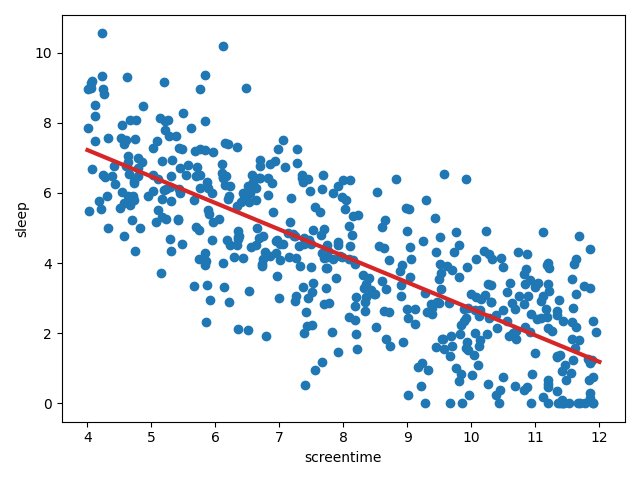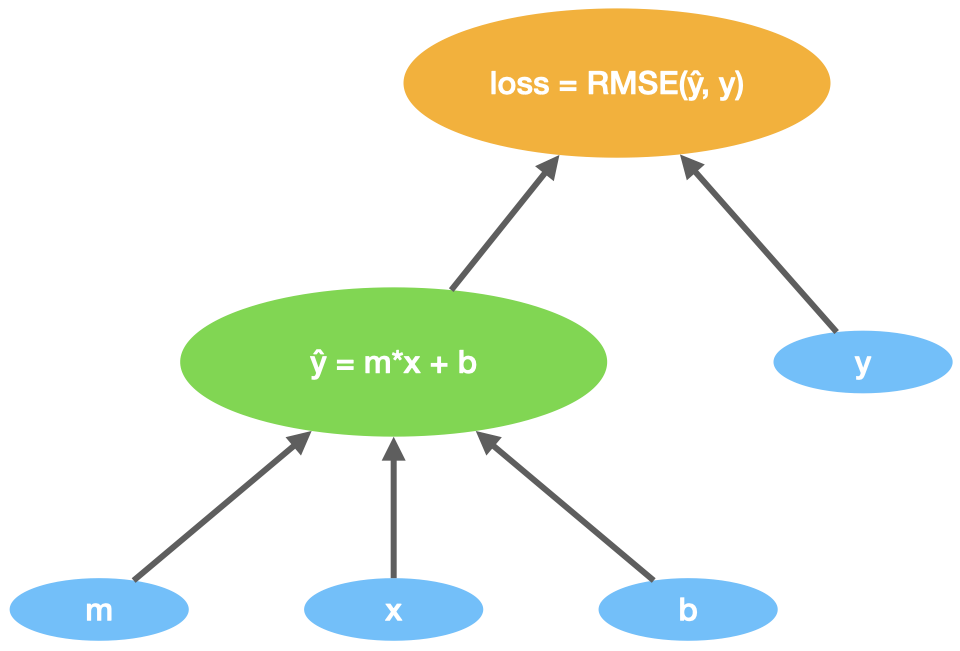# Screen Time Solution¶

# Initialize m and b
m = torch.tensor(, requires_grad=True, dtype=torch.float32)
b = torch.tensor(, requires_grad=True, dtype=torch.float32)

# Convert sleep and screentime to tensors
x = torch.from_numpy(screentime)
y = torch.from_numpy(sleep)

# Run 10K iterations
for i in range(10_000):

# Calculate yhat
yhat = m*x + b

# Calculate the loss
loss = torch.sqrt(torch.mean((yhat - y)**2))

# "Reverse mode differentiation"
loss.backward()

# Update m and b (gradient step)

# Zero out the gradients

print(f"(m, b) = ({m.item()}, {b.item()})")
# (m, b) = (-0.755, 11.241)

Plot
fig, ax = plt.subplots(layout='tight')
ax.scatter(screentime, sleep)
xlims = np.array(ax.get_xlim())
yvals = m.item() * xvals + b.item()
ax.plot(xlims, yvals, c='tab:red', linewidth=3)
ax.set_xlabel('screentime')
ax.set_ylabel('sleep')### Explanation¶

We'll flesh out the algorithm through a sequence of improving drafts.

# Initialize m and b
m = torch.tensor(, requires_grad=True, dtype=torch.float32)
b = torch.tensor(, requires_grad=True, dtype=torch.float32)

# Convert sleep and screentime to tensors
x = torch.from_numpy(screentime)
y = torch.from_numpy(sleep)

# Calculate yhat
yhat = m*x + b

# Calculate the loss
loss = torch.sqrt(torch.mean((yhat - y)**2))

# "Reverse mode differentiation"
loss.backward()

# Update m and b (gradient step)
m = m - 0.02*m.grad
b = b - 0.02*b.grad


1. Initialize m and b.

m = torch.tensor(, requires_grad=True, dtype=torch.float32)
b = torch.tensor(, requires_grad=True, dtype=torch.float32)

print(m) # tensor([0.], requires_grad=True)
print(b) # tensor([0.], requires_grad=True)


Here we initialize m as a tensor with a single float32 value, 0. Then we do the same for b.

Since we initialize m and b "from scratch", these are considered leaf tensors.

print(m.is_leaf)  # True
print(b.is_leaf)  # True

2. Convert sleep and screentime to tensors.

x = torch.from_numpy(screentime)
y = torch.from_numpy(sleep)


These are also leaf tensors.

print(x.is_leaf)  # True
print(y.is_leaf)  # True

3. Calculate yhat, the predicted sleep values based on the current m and b.

yhat = m*x + b

print(yhat)
# tensor([0., 0., ... 0., 0., 0.], dtype=torch.float64, grad_fn=<AddBackward0>)


Since yhat is created from existing tensors, it is not a leaf tensor.

print(yhat.is_leaf)  # False

4. Calculate the loss (root mean square error).

loss = torch.sqrt(torch.mean((yhat - y)**2)) # (1)!

print(loss)
# tensor(4.7595, dtype=torch.float64, grad_fn=<SqrtBackward0>)

1. Alternatively,

loss = (((yhat - y)**2).mean()).sqrt()

5. Calculate the gradient of the loss with respect to m and b.

Here's an oversimplified depiction of our computation graph.m, b, x, and y are leaves and loss is the root. Therefore, we can call loss.backward() , and PyTorch will automatically calculate the gradient of loss with respect to m and b.

loss.backward()



This tells us that increasing m should decrease the loss (and the same goes for b).

What about x, y, and yhat?

x and y
Even though x and y are leaves in the computational graph, their gradients won't be computed because their requires_grad attribute is turned off.

print(x.requires_grad)  # False


This is because x and y were created using torch.from_numpy() which does not turn on requires_grad.

This is the behavior we desire, but if you wanted x and y gradients to be calculated, you should create them like this.

x = torch.tensor(screentime, requires_grad=True)
y = torch.tensor(sleep, requires_grad=True)


yhat
yhat gradients are calculated but not retained. We know this because yhat.requires_grad is True and yhat.retains_grad is False. Furthermore, if you try to print yhat.grad, pytorch returns None with a warning.

print(yhat.grad) # None


UserWarning: The .grad attribute of a Tensor that is not a leaf Tensor is being accessed. Its .grad attribute won't be populated during autograd.backward(). If you indeed want the .grad field to be populated for a non-leaf Tensor, use .retain_grad() on the non-leaf Tensor...

Again, this is the behavior we desire, but if you wanted yhat gradients to be retained, call yhat.retain_grad() right after creating it.

yhat = m*x + b


Terminology note

In the context of neural networks, this is what people call backpropagation. But in the more general sense, this is known as reverse mode differentiation (aka reverse accumulation).

6. Update m and b (gradient step).

In step 5, we learned that loss should decrease if we make small increases to m and b. Here we update m and b accordingly, using a gradient step with alpha (aka "step size") equal to 0.02.

m = m - 0.02*m.grad
b = b - 0.02*b.grad

print(m.item(), b.item())  # 0.1241 0.0176


Important

The -= operator won't work here! (PyTorch doesn't allow in place operations on tensors that require gradients.)

m -= 0.02*m.grad


RuntimeError: a leaf Variable that requires grad is being used in an in-place operation.

Problem
This is the first iteration of our gradient descent algorithm. See what happens when we run the next iteration..

Gradient Descent Iteration 2
yhat = m*x + b
loss = torch.sqrt(torch.mean((yhat - y)**2))
loss.backward()
m = m - 0.02*m.grad  # <- error!


TypeError: The .grad attribute of a Tensor that is not a leaf Tensor is being accessed...

On this second iteration of gradient descent, it turns out m is not a leaf tensor! That's because, in step 6 above, m = m - 0.02*m.grad actually copies m's data into a new block of memory. You can see this by printing m's memory address before and after the update.

print(f'address before: {m.data_ptr()}')
m = m - 0.02*m.grad

# address before: 94445597513088
# address after:  94445597510720


We'll fix this in Draft 2 (link above).

# Initialize m and b
m = torch.tensor(, requires_grad=True, dtype=torch.float32)
b = torch.tensor(, requires_grad=True, dtype=torch.float32)

# Convert sleep and screentime to tensors
x = torch.from_numpy(screentime)
y = torch.from_numpy(sleep)

# Calculate yhat
yhat = m*x + b

# Calculate the loss
loss = torch.sqrt(torch.mean((yhat - y)**2))

# "Reverse mode differentiation"
loss.backward()

# Update m and b (gradient step)


Here we've changed

m = m - 0.02*m.grad
b = b - 0.02*b.grad


to

with torch.no_grad():


in order to update m and b in place as opposed to copying the tensors.

torch.no_grad is a context manager that temporarily disables gradient calculation. This allows us to use in place operators like -= and +=.

Info

PyTorch prevents you from using in place operators on tensors with requires_grad == True, but you can use them on tensors with requires_grad == False.

Alternatively, we could directly access and update the underlying data using the Tensor.data attribute.

m.data = m - 0.02*m.grad
b.data = b - 0.02*b.grad

Problem
We've only implemented one iteration of gradient descent. We need to implement more iterations!

# Initialize m and b
m = torch.tensor(, requires_grad=True, dtype=torch.float32)
b = torch.tensor(, requires_grad=True, dtype=torch.float32)

# Convert sleep and screentime to tensors
x = torch.from_numpy(screentime)
y = torch.from_numpy(sleep)

# Run 10K iterations
for i in range(10_000):

# Calculate yhat
yhat = m*x + b

# Calculate the loss
loss = torch.sqrt(torch.mean((yhat - y)**2))

# "Reverse mode differentiation"
loss.backward()

# Update m and b (gradient step)


Here we've wrapped the gradient descent process into a for loop that runs 10,000 iterations.

Problem
Let's inspect the first 10 iterations by inserting a informative print statement in the loop.

# Initialize m and b
m = torch.tensor(, requires_grad=True, dtype=torch.float32)
b = torch.tensor(, requires_grad=True, dtype=torch.float32)

# Convert sleep and screentime to tensors
x = torch.from_numpy(screentime)
y = torch.from_numpy(sleep)

# Run 10K iterations
for i in range(10_000):

# Calculate yhat
yhat = m*x + b

# Calculate the loss
loss = torch.sqrt(torch.mean((yhat - y)**2))

# "Reverse mode differentiation"
loss.backward()

# Print stuff
if i < 10:
print(
f"iteration {i}: "
f"(m, b) = ({round(m.item(), 3)}, {round(b.item(), 3)}), "
f"loss = {round(loss.item(), 3)}"
)

# Update m and b (gradient step)

iteration 0: (m, b) = (0.0, 0.0),     (m.grad, b.grad) = (-6.635, -0.917),  loss = 5.659
iteration 1: (m, b) = (0.133, 0.018), (m.grad, b.grad) = (-12.49, -1.77),   loss = 4.81
iteration 2: (m, b) = (0.383, 0.054), (m.grad, b.grad) = (-15.369, -2.338), loss = 3.642
iteration 3: (m, b) = (0.69, 0.1),    (m.grad, b.grad) = (-12.287, -2.216), loss = 3.666
iteration 4: (m, b) = (0.936, 0.145), (m.grad, b.grad) = (-6.342, -1.708),  loss = 4.839
iteration 5: (m, b) = (1.062, 0.179), (m.grad, b.grad) = (0.346, -1.088),   loss = 5.663
iteration 6: (m, b) = (1.056, 0.201), (m.grad, b.grad) = (7.018, -0.471),   loss = 5.63
iteration 7: (m, b) = (0.915, 0.21),  (m.grad, b.grad) = (12.883, 0.026),   loss = 4.751
iteration 8: (m, b) = (0.658, 0.21),  (m.grad, b.grad) = (15.652, 0.108),   loss = 3.582
iteration 9: (m, b) = (0.344, 0.207), (m.grad, b.grad) = (12.409, -0.497),  loss = 3.668


Notice how the parameters change wildly from iteration to iteration. This indicates that something's not quite right..

It turns out that PyTorch accumulates gradients by default!

# Initialize m and b
m = torch.tensor(, requires_grad=True, dtype=torch.float32)
b = torch.tensor(, requires_grad=True, dtype=torch.float32)

# Convert sleep and screentime to tensors
x = torch.from_numpy(screentime)
y = torch.from_numpy(sleep)

# Run 10K iterations
for i in range(10_000):

# Calculate yhat
yhat = m*x + b

# Calculate the loss
loss = torch.sqrt(torch.mean((yhat - y)**2))

# "Reverse mode differentiation"
loss.backward()

# Print stuff
if i < 10:
print(
f"iteration {i}: "
f"(m, b) = ({round(m.item(), 3)}, {round(b.item(), 3)}), "
f"loss = {round(loss.item(), 3)}"
)

# Update m and b (gradient step)

# Zero out the gradients


Because PyTorch accumulates gradients by default, we have to manually "zero out the gradients" by setting them to None.

m.grad = None


Now, the first 10 gradient descent iterations appear more stable.

iteration 0: (m, b) = (0.0, 0.0), (m.grad, b.grad) = (-6.635, -0.917), loss = 5.659
iteration 1: (m, b) = (0.133, 0.018), (m.grad, b.grad) = (-5.855, -0.853), loss = 4.81
iteration 2: (m, b) = (0.25, 0.035), (m.grad, b.grad) = (-4.765, -0.755), loss = 4.17
iteration 3: (m, b) = (0.345, 0.051), (m.grad, b.grad) = (-3.485, -0.63), loss = 3.763
iteration 4: (m, b) = (0.415, 0.063), (m.grad, b.grad) = (-2.296, -0.506), loss = 3.553
iteration 5: (m, b) = (0.461, 0.073), (m.grad, b.grad) = (-1.409, -0.41), loss = 3.463
iteration 6: (m, b) = (0.489, 0.081), (m.grad, b.grad) = (-0.832, -0.346), loss = 3.429
iteration 7: (m, b) = (0.506, 0.088), (m.grad, b.grad) = (-0.48, -0.306), loss = 3.416
iteration 8: (m, b) = (0.515, 0.094), (m.grad, b.grad) = (-0.27, -0.282), loss = 3.41
iteration 9: (m, b) = (0.521, 0.1), (m.grad, b.grad) = (-0.147, -0.268), loss = 3.407


Notice how the parameters change more smoothly instead of wildly bouncing around as they did in the previous draft.

At the end of 10,000 iterations, we get m = -0.755 and b = 11.241.